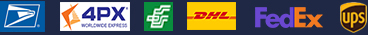•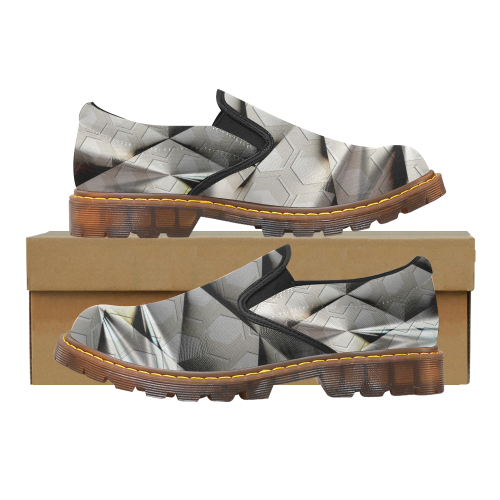•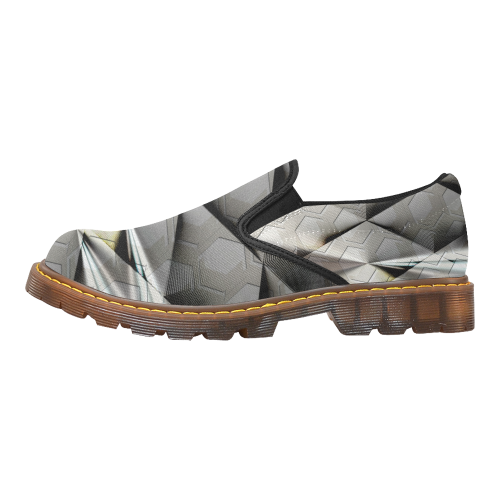•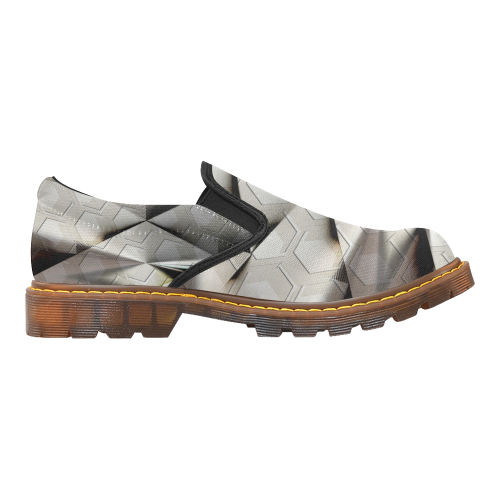•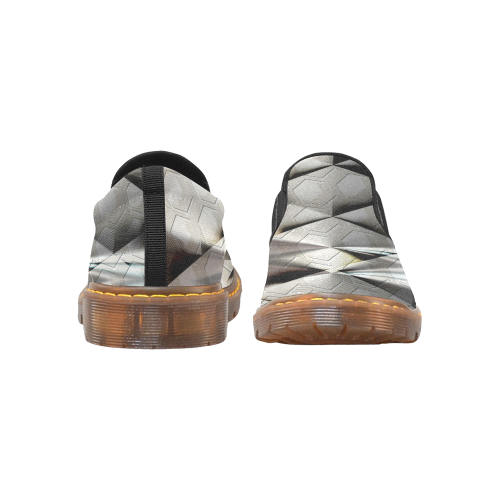### Men's Slip-On Loafer (Model 12031)

\$39.99
Retail Price \$69.99

Shipping Included

•   Black
•   White

EUR39 = US7 =UK6.5 =256mm =10.08inch

EUR40 = US8 =UK7=261mm =10.28inch

EUR41 = US8.5 =UK7.5=263mm =10.35inch

EUR42 = US9 =UK8= 268mm = 10.55inch

EUR43 = US10 =UK8.5=275mm = 10.83inch
EUR44 = US10.5 =UK9 = 281mm = 11.18inch
EUR45 = US11 =UK10=286mm = 11.26inch
EUR46 = US12 =UK11 = 293mm = 11.54inch

Payment methods we accpet: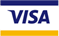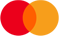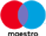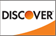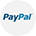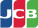We deliver with: